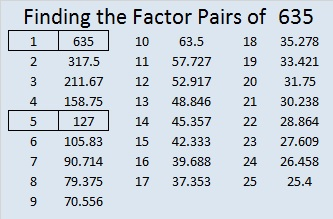# 635 Some Multiplication Facts to Know Backwards and Forwards

Something amazing happens in the multiplication table when an even number is multiplied by 6.

Something just as amazing (but backwards) happens in the multiplication table when a multiple of 3 is multiplied by 7.

Here is a graphic that may be helpful for students memorizing some of those 6 and 7 multiplication facts. It is meant to be read from left to right and contains some cool coincidences:Knowing those 6 and 7 multiplication facts will help any student know the multiplication table backwards and forwards!

While it isn’t necessary to memorize the following number facts, some of the patterns in the graphic above continue with them:

• 10 + 5 = 15, and 15 x 7 = 105
• 12 + 6 = 18, and 18 x 7 = 126
• 14 + 7 = 21, and 21 x 7 = 147
• 16 + 8 = 24, and 24 x 7 = 168
• 18 + 9 = 27, and 27 x 7 = 189

———————————————————

What makes 635 an interesting number?

635 is the sum of the nine prime numbers from 53 to 89.

It is also the sum of the thirteen prime numbers from 23 to 73.

635 can be written as the sum of 5 consecutive numbers:

125 + 126 + 127 + 128 + 129 = 635.

635 is a part of exactly 4 Pythagorean triples. Which factors of 635 are greatest common factors for the non-primitive triples?

• 635 is the hypotenuse of the Pythagorean triple 381-508-635
• 635 is the short leg in the triple 635-1524-1650
• 635 is the short leg in the triple 635-40320-40325
• 635 is the short leg in the primitive triple 635-201612-201613

———————————————————————–

• 635 is a composite number.
• Prime factorization: 635 = 5 x 127
• The exponents in the prime factorization are 1 and 1. Adding one to each and multiplying we get (1 + 1)(1 + 1) = 2 x 2 = 4. Therefore 635 has exactly 4 factors.
• Factors of 635: 1, 5, 127, 635
• Factor pairs: 635 = 1 x 635 or 5 x 127
• 635 has no square factors that allow its square root to be simplified. √635 ≈ 25.199206.